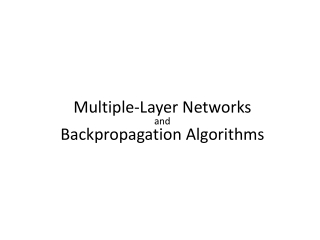Download PresentationM ultiple - L ayer N etworks and Backpropagation Algorithms

Loading in 2 Seconds...

# M ultiple - L ayer N etworks and Backpropagation Algorithms

Download Presentation## M ultiple - L ayer N etworks and Backpropagation Algorithms

- - - - - - - - - - - - - - - - - - - - - - - - - - - E N D - - - - - - - - - - - - - - - - - - - - - - - - - - -
##### Presentation Transcript

1. Multiple-LayerNetworksandBackpropagationAlgorithms

2. Multiple-LayerNetworksandBackpropagationAlgorithms Backpropagation is the generalization of the Widrow-Hoff learning rule to multiple-layer networks and nonlinear differentiable transfer functions. Input vectors and the corresponding target vectors are used to train a network until it can approximate a function, associate input vectors with specific output vectors, or classify input vectors in an appropriate way as defined by you.

3. Architecture This section presents the architecture of the network that is most commonly used with the backpropagation algorithm – the multilayer feedforward network

4. An elementary neuron with R inputs is shown below. Each input is weighted with an appropriate w. The sum of the weighted inputs and the bias forms the input to the transfer function f. Neurons can use any differentiable transfer function f to generate their output. Architecture Neuron Model

5. Multilayer networks often use thelog-sigmoid transfer function logsig. The function logsig generates outputs between 0 and 1 as the neuron's net input goes from negative to positive infinity ArchitectureNeuron ModelTransfer Functions (ActivitionFunction)

6. Alternatively, multilayer networks can use the tan-sigmoid transfer function-tansig. The function logsig generates outputs between-1and +1 as the neuron's net input goes from negative to positive infinity ArchitectureNeuron ModelTransfer Functions (ActivitionFunction)

7. A single-layer network of S logsig neurons having R inputs is shown below in full detail on the left and with a layer diagram on the right. Architecture Feedforward Network

8. Feedforward networks often have one or more hidden layers of sigmoid neurons followed by an output layer of linear neurons. Multiple layers of neurons with nonlinear transfer functions allow the network to learn nonlinear and linear relationships between input and output vectors. The linear output layer lets the network produce values outside the range -1 to +1.On the other hand, if you want to constrain the outputs of a network (such as between 0 and 1), then the output layer should use a sigmoid transfer function (such as logsig). Architecture Feedforward Network

9. The following slides describes teaching process of multi-layer neural network employing backpropagation algorithm. To illustrate this process the three layer neural network with two inputs and one output,which is shown in the picture below, is used:  Learning Algorithm:Backpropagation

10. Each neuron is composed of two units. First unit adds products of weights coefficients and input signals. The second unit realise nonlinear function, called neuron transfer (activation) function. Signal e is adder output signal, and y = f(e) is output signal of nonlinear element. Signal y is also output signal of neuron.  Learning Algorithm:Backpropagation

11. To teach the neural network we need training data set. The training data set consists of input signals (x1 and x2 ) assigned with corresponding target (desired output) z. The network training is an iterative process. In each iteration weights coefficients of nodes are modified using new data from training data set. Modification is calculated using algorithm described below: Each teaching step starts with forcing both input signals from training set. After this stage we can determine output signals values for each neuron in each network layer. Learning Algorithm:Backpropagation

12. Pictures below illustrate how signal is propagating through the network, Symbols w(xm)n represent weights of connections between network input xm and neuron n in input layer. Symbols yn represents output signal of neuron n. Learning Algorithm:Backpropagation

13. Learning Algorithm:Backpropagation

14. Learning Algorithm:Backpropagation

15. Propagation of signals through the hidden layer. Symbols wmn represent weights of connections between output of neuron m and input of neuron n in the next layer.  Learning Algorithm:Backpropagation

16. Learning Algorithm:Backpropagation

17. Learning Algorithm:Backpropagation

18. Propagation of signals through the output layer. Learning Algorithm:Backpropagation

19. In the next algorithm step the output signal of the network y is compared with the desired output value (the target), which is found in training data set. The difference is called error signal d of output layer neuron Learning Algorithm:Backpropagation

20. The idea is to propagate error signal d (computed in single teaching step) back to all neurons, which output signals were input for discussed neuron.  Learning Algorithm:Backpropagation

21. The idea is to propagate error signal d (computed in single teaching step) back to all neurons, which output signals were input for discussed neuron.  Learning Algorithm:Backpropagation

22. The weights' coefficients wmn used to propagate errors back are equal to this used during computing output value. Only the direction of data flow is changed (signals are propagated from output to inputs one after the other). This technique is used for all network layers. If propagated errors came from few neurons they are added. The illustration is below:  Learning Algorithm:Backpropagation

23. When the error signal for each neuron is computed, the weights coefficients of each neuron input node may be modified. In formulas below df(e)/de represents derivative of neuron activation function (which weights are modified). Learning Algorithm:Backpropagation

24. When the error signal for each neuron is computed, the weights coefficients of each neuron input node may be modified. In formulas below df(e)/de represents derivative of neuron activation function (which weights are modified). Learning Algorithm:Backpropagation

25. When the error signal for each neuron is computed, the weights coefficients of each neuron input node may be modified. In formulas below df(e)/de represents derivative of neuron activation function (which weights are modified). Learning Algorithm:Backpropagation